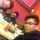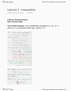# MATA33H3 Midterm: Complete And Comprehensive 34-Page Study Guide - Winter 2016

388 views34 pagespinkox631 and 3 others unlocked3
Verified Note
3 documents

## Document Summary

Topic included: linear inequalities in two variables, linear programming, multiple optimal solutions. It is the study of a geometric approach in order to optimize a linear function of two variables whose domain is a certain subset of a plane defined by some inequality. 3x + 2y 6 x y > 10. X + 2y = 4 (linear equations are also possible) X + 2y 4 x y < 3 x 0 (also = 1x + 0y 0) y 0 (both are known as non-negativity conditions ) Given a linear inequality the solution is the satisfied set is all (x,y) points in the plane, that satisfy the inequality (i. e. makes the inequality true) Solve the inequality: 3x +2y 6 (0,0) , (1,0) , (0,2) satisfy the inequality. Figure. 1. 1 a graphical visual of the inequality, shaded in red is the desired region. Not that the underlying line 3x + 2y = 6 is also included in the inequality.